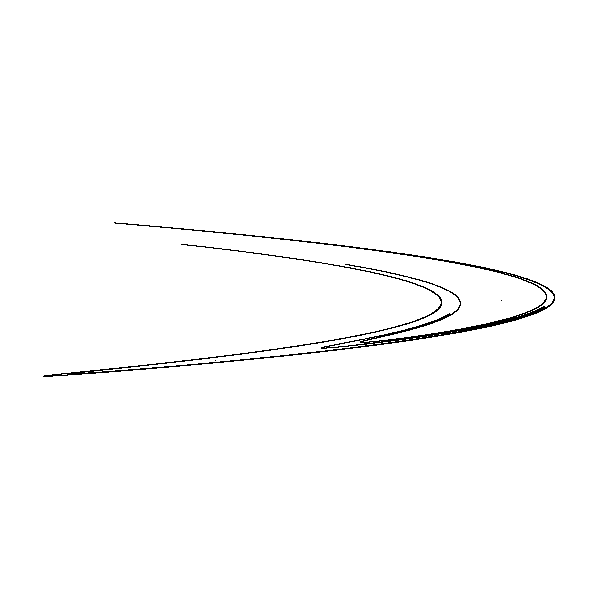# Henon fractal in generativepy

Martin McBride
2021-12-17

The Henon fractal is a strange attractor that is usually rendered in black and white (similar to the gingerbread man fractal). It is worth reading the Tinkerbell fractal article before tackling this fractal.

The Henon fractal is not the most visually exciting fractal, but its relative simplicity means that it has been studied extensively, so it is worth knowing about.

We are basing this fractal on the black and white Tinkerbell.

## Henon equations

The fractal equations for Henon are:

xnext = 1 - A*x*x + y
ynext = B*x


The classic Henon attaractor has:

A = 1.4
B = 0.3


Suitable initial values are:

x = 0.01  # Initial value
y = 0.01  # Initial value


Here is the result:## The code

Here is the full code for the image above:

from generativepy.bitmap import Scaler
from generativepy.nparray import make_nparray

MAX_COUNT = 100000
A = 1.4
B = 0.3

def paint(image, pixel_width, pixel_height, frame_no, frame_count):
scaler = Scaler(pixel_width, pixel_height, width=3, startx=-1.5, starty=-1.5)

x = 0.01
y = 0.01
for i in range(MAX_COUNT):
x, y = 1 - A*x*x + y, B*x
px, py = scaler.user_to_device(x, y)
if 0 <= px < pixel_width and 0 <= py < pixel_height:
image[py, px] = 0

make_nparray('henon.png', paint, 600, 600, channels=1)


This code is available on github in blog/fractals/henon.py.

This code is similar to the Tinkerbell implementation, the main changes are the formula used and the Scaler range.

In addition, it includes a range check on the pixel before plotting it:

        if 0 <= px < pixel_width and 0 <= py < pixel_height:
image[py, px] = 0


This is required when we zoom in on the fractal, to avoid attempting to plot pixels that are outside the viewing area (see below).

## Zooming in

We can zoom in on the right hand tip of the fractal by altering the Scaler parameters:

    scaler = Scaler(pixel_width, pixel_height, width=.5, startx=0.8, starty=-0.25)


This creates the following image:This reveals more detail in the curve, as it splits into several separate curves. Zooming in further will reveal that each of those curves is also composed of several other curves.

If you wish to zoom in further, it will be necessary to increase the MAX_COUNT value, otherwise there will not be enough points to properly show the curve.